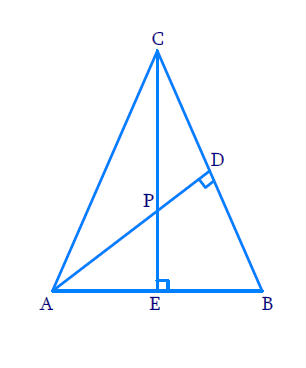# Ex.6.3 Q7 Triangles Solution - NCERT Maths Class 10

Go back to  'Ex.6.3'

## Question

In Figure, altitudes $$AD$$ and $$CE$$ of $$\Delta ABC$$ intersect each other at the point .Show that:

(i)  $$\Delta AEP\sim{\ }\Delta CDP$$

(ii)  $$\Delta ABD\sim{\ }\Delta CBE$$

(iii)  $$\Delta AEP\sim\Delta ADB$$

(iv)  $$\Delta \text{ }PDC\sim\Delta BEC$$

DiagramVideo Solution
Triangles
Ex 6.3 | Question 7

## Text Solution

(i) Reasoning:

If two angles of one triangle are respectively equal to two angles of another triangle, then the two triangles are similar.

This may be referred to as the $$AA$$ similarity criterion for two triangles.

Steps:

In $$\Delta AEP$$ and $$\Delta CDP$$

\begin{align}&\angle AEP = \angle CDP = {90^\circ }\\&\left[ \begin{array}{l}\therefore CE \bot AB\,\,{\rm{and}}{\mkern 1mu} {\mkern 1mu} {\rm{AD}} \bot {\rm{BC}};{\mkern 1mu} \,{\rm{altitudes}}\end{array} \right]\\\\&\angle APE = \angle CPD\\&\;\;\left[ \begin{array}{l}{\rm{Vertically}}\,{\rm{opposite}}{\mkern 1mu} \,{\rm{angles}}\end{array} \right]\\\\& \Rightarrow \Delta AEP\sim\Delta CPD\\&\qquad\left[ {{\text{AA Criterion}}} \right]\end{align}

(ii) Reasoning:

If two angles of one triangle are respectively equal to two angles of another triangle, then the two triangles are similar.

This may be referred to as the $$AA$$ similarity criterion for two triangles.

Steps:

In $$\Delta ABD\,{\rm {and}}\,\Delta CBE$$

\begin{align}\angle ADB &= \angle CEB = {90^\circ }\\\angle ABD &= \angle CBE\\ &[ \text{Common angle}]\\\\ \Rightarrow \,\Delta ABD &\sim \Delta CBE\\ [AA&\text{ Criterion}]\end{align}

(iii) Reasoning:

If two angles of one triangle are respectively equal to two angles of another triangle, then the two triangles are similar.

This may be referred to as the $$AA$$ similarity criterion for two triangles.

Steps:

In $$\Delta\rm{ A E P}$$ and $$\Delta\rm{ A D B}$$

\begin{align}\angle AEP &= \angle ADB = {90^\circ }\\\angle PAE &\!=\! \angle BAD \\ &[ \text{Common Angle}]\\\\ \Rightarrow \quad \Delta AEP &\sim \Delta ADB\end{align}

(iv) Reasoning:

If two angles of one triangle are respectively equal to two angles of another triangle, then the two triangles are similar.

This may be referred to as the $$AA$$ similarity criterion for two triangles.

Steps:

In $$\Delta P D C \,{\rm{and}}\, \Delta B E C$$

\begin{align}\angle PDC &= \angle BEC = {90^\circ }\\\angle PCD &= \angle BCE \\& [\text{Common Angle}]\\\\ \Rightarrow \quad\Delta PDC &- \Delta BEC\end{align}

Learn from the best math teachers and top your exams

• Live one on one classroom and doubt clearing
• Practice worksheets in and after class for conceptual clarity
• Personalized curriculum to keep up with school